# ML Wiki

## Workflow Patterns in Workflow Nets

### Sequence

First task $a$, then task $b$

•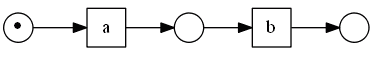### Parallel Split

Tasks $b$ and $c$ are processed in parallel

• this construction is also called "AND-split"
•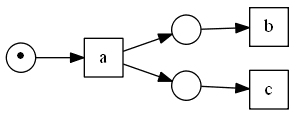### Synchronization

Do $d$ only after both $b$ and $c$ are completed

• this is a synchronization between two parallel processes
• this construction is also called "AND-join"
•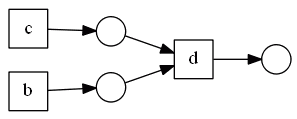### Parallel Routing

A combination of Parallel Split and Synchronization

•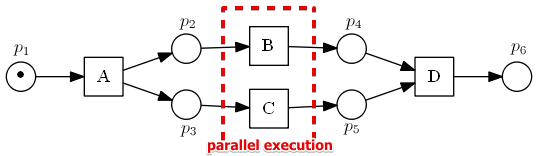### Exclusive Choice

after $a$, do $b$ or $c$

•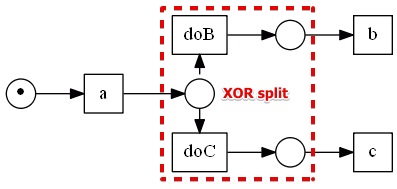• choice in this case depends on the results of the activity $a$
• here both doA and doB will be active, but we want to fire only one of them, based on the activity $a$

### Simple Merge

perform $d$ after $b$ or $c$ finishes

•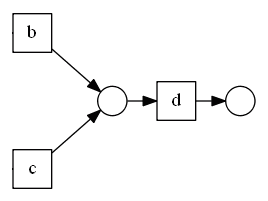### Alternative Routing

a combination of Exclusive Choice and Simple Merge

•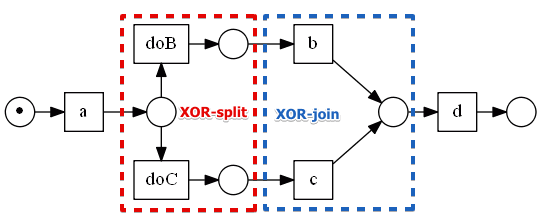### Deferred Choice

When the choice is deferred to the point when we execute something

• for Petri Nets, visually looks identical to Exclusive Choice
•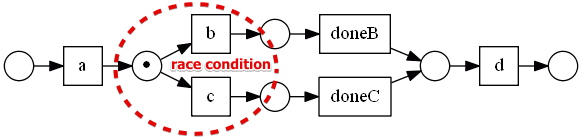Deferred Choice vs Exclusive Choice

• in Exclusive Choice after execution of $a$ you already know what activity to run
• but for the deferred choice there's a race condition between the activities